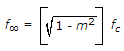# Electronics and Communication Engineering - Networks Analysis and Synthesis

1.

A capacitor is considered to be completely charged in __________ time constant.

 A. one B. three C. five D. eight

Explanation:

No answer description available for this question. Let us discuss.

2.

For an m-derived high pass filterA. True B. False

Explanation:

No answer description available for this question. Let us discuss.

3.

Consider the following statements associated with two port networks.

1. Z12 = Z21
2. Y12 = Y21
3. h12 = h21
4. AD - BC = 1
Which one of the statement given above are correct?

 A. 1, 2, 3 B. 2, 3, 4 C. 1, 3, 4 D. 1, 2, 4

Explanation:

No answer description available for this question. Let us discuss.

4.

Assertion (A): The number of basic loops is equal to number of links.

Reason (R): The graph theory helps in choosing independent variables in circuit analysis.

 A. Both A and R are true and R is correct explanation of A B. Both A and R are true and R is not the correct explanation of A C. A is true but R is false D. A is false but R is true

Explanation:

No answer description available for this question. Let us discuss.

5.

In an R-L-C circuit, v(t) = 20 sin (314 t + 5 p/6) and i(t) = 10 sin (314 t + 2p/3). The p.f of the circuit is